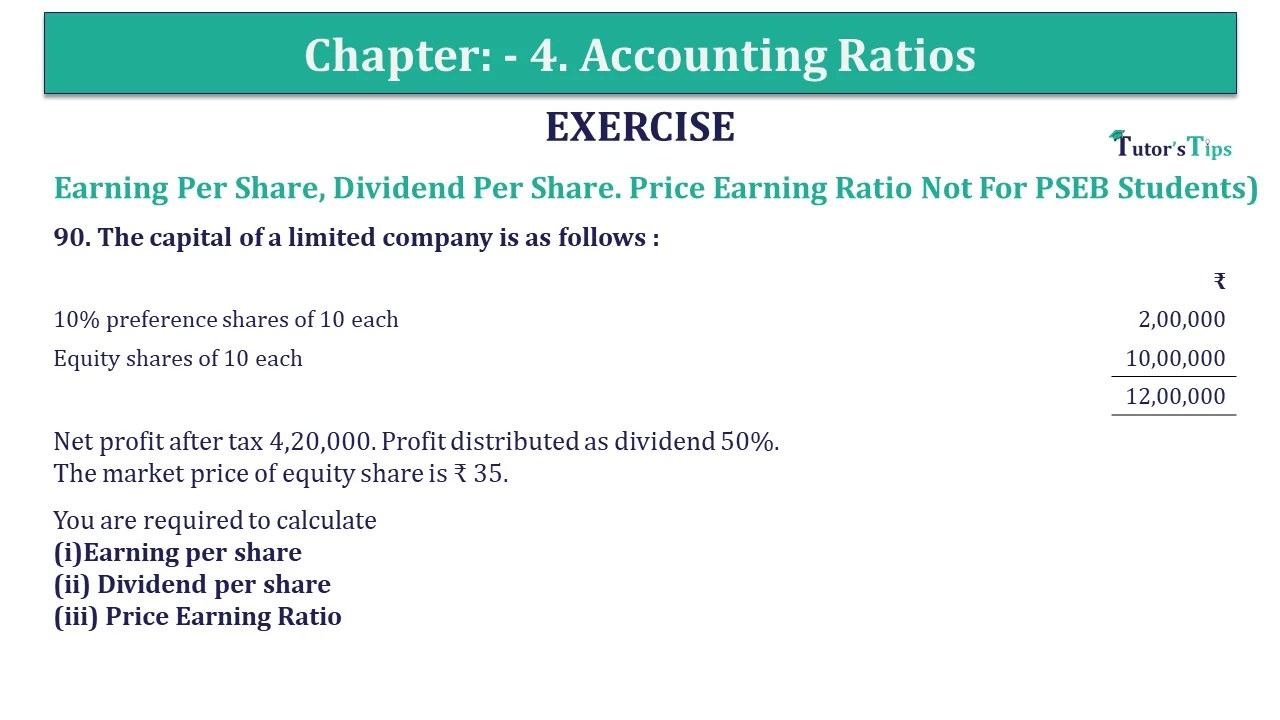# Question 90 Chapter 4 of +2-B – USHA Publication 12 ClassQ-90- CH-4 Book 2 - Usha Pub. +2 Book 2020 - Solution

Question 90 Chapter 4 of +2-B

Earning Per Share, Dividend Per Share. Price Earning Ratio

90. The capital of a limited company is as follows :

 ₹ 10% preference shares of 10 each 2,00,000 Equity shares of 10 each 10,00,000 12,00,000

Net profit after tax 4,20,000. Profit distributed as dividend 50%.
The market price of equity share is ₹ 35.

You are required to calculate
(i)Earning per share
(ii) Dividend per share
(iii) Price Earning Ratio

## The solution of Question 90 Chapter 4 of +2-B: –

 Earnings per Share = Net profit after Interest and Tax Preference Dividend Number of Equity Shares = = ₹ 4,00,000 1,00,000 = ₹ 4
 Dividend Per share = Profit distributed as equity share Number of equity shares = = ₹ 2,00,000 1,00,000 = ₹ 2

 Price Earning Ratio = Market price per share Earning per share = = ₹ 35 ₹ 4 = 8.75 times

 Preference Dividend = 10% Preference share = 10 X ₹ 2,00,000 100 = ₹ 20,000 Net profit preference Dividend = Profit after tax – Preference Dividend = ₹ 4,20,000 – ₹ 20,000 = ₹ 4,00,000

Also, Check out the solved question of previous Chapters: –